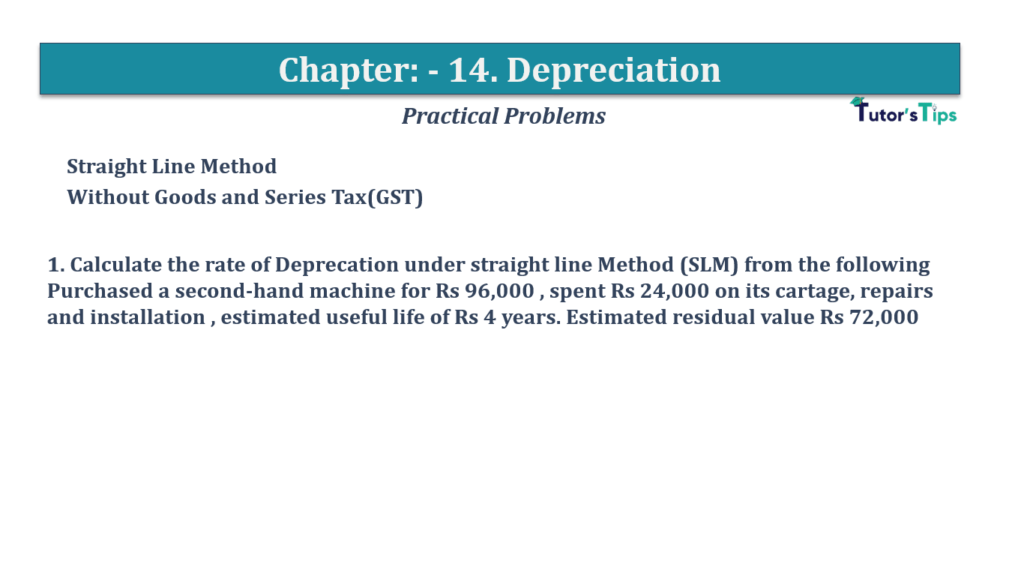# Question No 1 Chapter No 14 – T.S. Grewal 11 ClassQuestion No 1 Chapter No 14

Straight Line Method
Without Goods and Series Tax(GST)

1. Calculate the rate of Deprecation under straight-line Method (SLM) from the following Purchased a second-hand machine for Rs 96,000, spent Rs 24,000 on its cartage, repairs and installation, estimated useful life of Rs 4 years. Estimated residual value Rs 72,000

The solution of Question No 1 Chapter No 14: –

The method of calculating the Deprecation rate:

 Amount of Depreciation = Cost of assets- Estimated Realizable or Scrap value Number of years of expected useful life

Rate of Deprecation can be calculated with the help of following formula:

 Rate of Depreciation = Amount of Depreciation X100 Total Cost of Assets

Cost of assets = Cost of Machine + Installation cost 96,000+24,000 = 1,20,000
Number of years of expected useful life = 4 years
Estimated Retaliated or Scrap value = 72,000

 Amount of Depreciation = 1,20,000 – 72,000 = 12,000 4

Rate of Deprecation can be calculated with the help of following formula:

 Rate of Depreciation = 12,000 X100 1,20,000

=10%

Depreciation | Meaning | Methods | Examples

Comment if you have any question.

Also, Check out the solved question of previous Chapters: –

• Chapter No. 1 – Introduction to Accounting
• Chapter No. 2 – Basic Accounting Terms
• Chapter No. 3 – Theory Base of Accounting, Accounting Standards and International Financial Reporting Standards(IFRS)
• Chapter No. 4 – Bases of Accounting
• Chapter No. 5 – Accounting Equation
• Chapter No. 6 – Accounting Procedures – Rules of Debit and Credit
• Goods and Services Tax(GST)
• Chapter No. 7 – Origin of Transactions – Source Documents and Preparation of Vouchers
• Chapter No. 8 – Journal
• Chapter No. 9 – Ledger
• Chapter No. 10 – Special Purpose Books I – Cash Book
• Chapter No. 11 – Special Purpose Books II – Other Books
• Chapter No. 12 – Bank Reconciliation Statement
• Chapter No. 13 – Trial Balance
• Chapter No. 14 – Depreciation
• Chapter No. 15 – Provisions and Reserves
• Chapter No. 16 – Accounting for Bills of Exchange
• Chapter No. 17 – Rectification of Errors
• Chapter No. 18 – Financial Statements of Sole Proprietorship
• Chapter No. 19 – Adjustments in preparation of Financial Statements
• Chapter No. 20 – Accounts from incomplete Records – Single Entry System
• Chapter No. 21 – Computers in Accounting
• Chapter No. 22 – Accounting Software – Tally
• Chapter No. 5 – Accounting Equation
• Chapter No. 6 – Accounting Procedures – Rules of Debit and Credit
• Goods and Services Tax(GST)
• Chapter No. 8 – Journal
• Chapter No. 9 – Ledger
• Chapter No. 10 – Special Purpose Books I – Cash Book

This site uses Akismet to reduce spam. Learn how your comment data is processed.